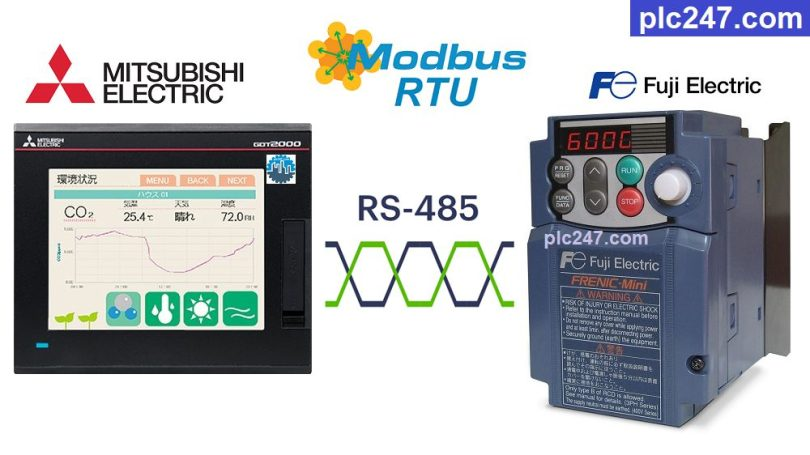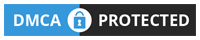# Mitsubishi HMI “Modbus RTU” Fuji Frenic TutorialWritten by

Hello everyone, continuing to use HMI Mitsubishi GT2505-VTBD to control various devices through Modbus RTU communication protocol, today plc247.com continues to use it to control Fuji Frenic inverters.

Fuji Frenic inverter model used is Fuji Frenic Mini (Fuji Eco series, Multi analog control). Models that have been produced for a long time have an RS485 card, while current models have a built-in RS485 port.

#### HMI Mitsubishi & Fuji Frenic “Wiring Diagram”

If the communication distance between the HMI and the inverter is far (>50m), it is necessary to use an additional 120Ω terminal resistor to make the connection stable.

#### Fuji Frenic “Modbus RTU” Parameters Setting

In order for HMI GT2505-VTBD to control and monitor the Fuji Frenic inverter, in addition to the basic parameters of the inverter (frequency, current, voltage…) we need to install additional communication parameters as follows:

+ H30 = 3 (VFD Control via RS485 Port)

+ Y01 = 1 (Slave Address)

+ Y02 = 0

+ Y03 = 2

+ Y04 = 2 (9600bps)

+ Y05 = 0 (Data: 8bit)

+ Y06 = 1 (Parity Check: Even)

+ Y07 = 1 (Stop Bit: 1)

+ Y10 = 0 (Modbus-RTU Selection)

+ Y99 = 0

• Functions Mapping
##### Note: Mitsubishi GOT2000 Holding Registers Address = “VFD Address (Dec) + 400001“

+ Frequency reference = S(07H) + 05 = 705 (hexa) = 1797 (dec)
>>> Frequency Setting Address = 1797 + 400001 = 401798 (dec)

+ Operation command = S(07H) + 06 = 706 (hexa) = 1798 (dec)
>>> Motor Control Address = 1798 + 400001 = 401799 (dec)

• Set Word “401799” = 1 >>> Forward Run
= 2 >>> Reverse Run
= 0 >>> Stop Run

+ Output frequency = M(08H) + 09 = 809 (hexa) = 2057 (dec)
>>> Frequency Output Address = 2057 + 400001 = 402058 (dec)

+ Output current = M(08H) + 11 = 811 (hexa) = 2059 (dec)
>>> Current Output Address = 2059 + 400001 = 402060 (dec)

+ Output voltage = M(08H) + 12 = 812 (hexa) = 2060 (dec)
>>> Current Output Address = 2060 + 400001 = 402061 (dec)

#### Mitsubishi GT2505-VTBD Modbus Configuration

How have you set up the communication parameters for the Fuji Frenic inverter, the settings for the Mitsubishi HMI are exactly the same, the HMI and the inverter can communicate.

### HMI Programming

+ Interface Design

+ Frequency Setting Command

+ Forward Run Command

+ Reverse Run Command

+ VFD Stop Command

### Project Video Tutorial

======

Related Software and Documents